### A Tutorial on The Basics of Sound: Notes, Figures and Problems with Solutions

Target Audience: High School Students, College Freshmen and Sophomores, students preparing for the International Baccalaureate (IB), AP Physics B, AP Physics C, A Level, Singapore/GCE A-Level;

### This might also be helpful in studying topics required by Common Core Physics.

This compilation of notes has been prepared by Anirud Thyagarajan of IIT Kharagpur

SOUND WAVES

a.) The sound waves can also be called as elastic waves and medium is needed for their propagation. Their velocity is different for different media.

b.) The velocity of sound waves depends on the property of inertia and coefficient of elasticity of that medium.

c.) In this type of motion the energy is transferred from one point to other by the propagation of disturbance in the medium. The motion of disturbance is called the wave motion.

d.) In simple harmonic wave the particles of the medium executes simple harmonic motion. Each particle of the medium executes simple harmonic motion at all the times, though the phase of its vibration depends on its position and time.

On the basis of vibration of the particle waves are classified in two types:

### (b)   Longitudinal waves

(a) Transverse waves:

The particles of medium vibrate in a direction perpendicular to the direction of propagation of wave. Their polarization is possible. Example: Vibration of string, the surface waves produced on the surface of solid and liquid.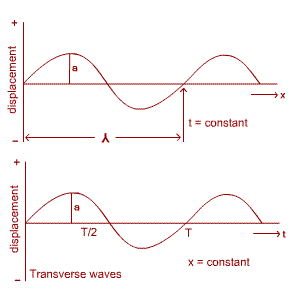Fig (1)

(b) Longitudinal waves:

Vibrations of the particles of the medium are in the direction of wave propagation wave proceeds in form of compression and rarefaction. At places of compression the pressure and density are maximum, while at places of rarefaction those are minimum.

Example: Sound waves, waves is gases, waves is solids.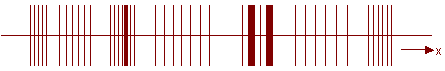Fig (2)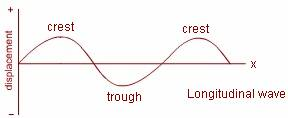### 2) Points related to Wave Motion:

1) Amplitude: The maximum displacement of a vibrating particle of the medium from the mean position.

2) Time period (T): The time taken by the particle to complete one vibration.

3) Frequency: The number of vibration in one second by the particle n = 1/T.

Angular Frequency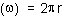4) Wavelength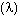: The distance between two consecutive particles vibrating in the same phase.

Or

The distance between two consecutive crests or trough.

Or

The distance between two consecutive compressions or rarefaction.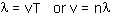Here v is the velocity of wave.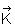is the wave propagation vector which is written as: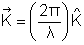,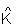being the unit vector along the direction of wave propagation then,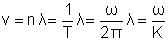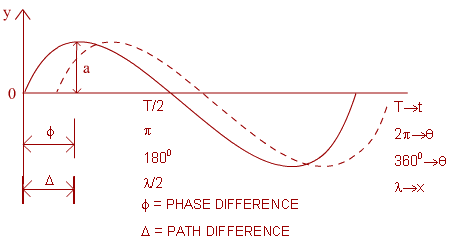Fig (3)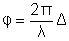### 3) Equations for the progressive Simple Harmonic Waves:

1) The equation of a wave moving along the direction of positive X-axis is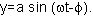Where ‘a’ is the amplitude,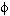is the phase difference and ‘y’ is the displacement of a particle.

2) The equation of a progressive wave moving along the direction of negative X-axis is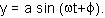3) The general equation of wave moving along X-axis is: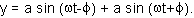4) Other equation for a progressive wave moving along the direction of positive X-axis is: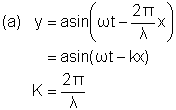Is propagation constant or wave vector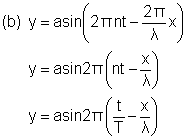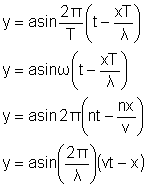Points to remember: Wave equation can be shown by any of the following relations: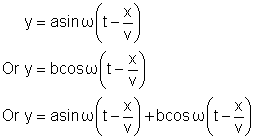All these waves are also called as ‘sinusoidal’ waves.

### 5) Intensity of waves, Energy Density:

1) Intensity (I): Amount of energy flowing per second from unit area of the medium in a direction perpendicular to the propagation of wave.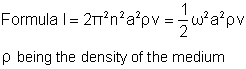2) For a given medium (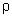and v are constants)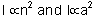3) Unit of Intensity: Joule/m2 second or watt/m2.

4) Energy density (E): Energy per unit volume of the medium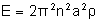5) For second waves the intensity obeys the inverse square law. If the distance between the source and the point of observation is ‘x’ then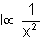### 6) Superposition of Waves:

1) If two or more waves propagate in a medium simultaneously, then the resultant displacement of the particle of the medium is given by the vector sum of displacements produced by the individual wave.

2) If the displacement produced by different waves are respectively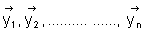Then the resultant displacement of particle is given by,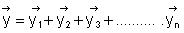3) Resultant displacement depends on

a) Amplitude

b) Phase difference

c) Frequencies

d) Direction of propagation of waves

4) Different phenomena occurring as a result of superposition of waves are

a) Interference

b) Beats

c) Stationary waves

d) Lissajous figures.

### 7) Interference:

1) As a result of superposition of two waves of same frequency traveling in the same direction simultaneously, the phenomenon of intensity of resultant wave becoming maximum and minimum is called as interference.

2) Equation of resultant wave formed by superposition of two waves: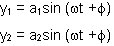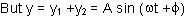Amplitude of the resultant wave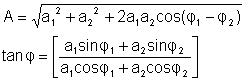3) Intensity of the resultant wave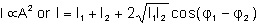I1 and I2 are separate intensities of the superposing waves.

4) Law of conservation of energy is obeyed in interference also, only the energy of the medium is redistributed.

### 8) Constructive and Destructive Interference:

1) When superposing waves are in the same phase then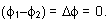The amplitude of the resultant waveIn this condition the interference is called ‘constructive interference’

2) For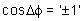if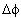is zero or even multiple of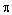;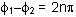, where n = 0, 1, 2, ----------------   or path difference of waves.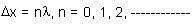3) Then in above condition, the intensity of resultant wave is maximum.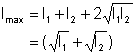4) If the interfering waves are in opposite phase then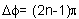, and path difference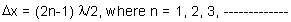In this condition the amplitude of the resultant wave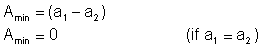Interference of this kind is called the ‘destructive interference’. In this condition the resultant intensity is also minimum.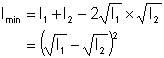5) Ratio of maximum intensity and minimum intensity of a wave is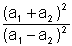6) To observe clear interference:

a) Phase difference of the waves must be fixed.

b) Amplitude of the waves must be equal.

### 9) Beats:

1) When two progressive sound waves of nearly equal frequencies superpose while traveling in the same direction, then the intensity of the resultant sound increases and decreases with time.

2) The increase and decrease (waxing and waning) of intensity of sound occurs with a regular interval. This regular waxing and waning of sound is called Beats. One decrease and one increase together make one BEAT.

3) Number of beats produced in one second is called to be beat frequency.

4) If the frequencies of the waves are m and n (m>n), then beat frequency = m-n. This difference must not be greater than 10Hz, otherwise beats are not heard.

5) Beat period = 1/ N = 1/ (m-n).

6) If the waves producing beats areThen the equation of the resultant wave is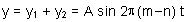And the amplitude of the wave is;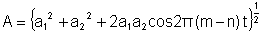### 10) APPLICATION OF BEATS:

2) In manufacturing the electronic oscillators of less and stable frequencies.

3) In detecting poisonous gases produced in mines.

4) In the tuning of musical instruments.

5) In determining unknown frequency of tuning fork: Let the known frequency of the tuning fork be ‘m’, unknown frequency be ‘n’ and the number of beats produced be ‘N’ while playing these two together.

(a) If by putting some wax on the arm of unknown frequency tuning fork:

If the beat frequency decreases then n = m+N.

If the beat frequency increases then n = m-N.

(b) If by putting wax on the arm of known frequency tuning fork:

If the beat frequency decreases, then n = m-N.

If the beat frequency increases, then n = m+N.

(c) If the arms of unknown frequency tuning fork are filled then:

If the beat frequency increase n = m+N.

If the beat frequency decrease n = m-N.

### 11) Reflection of sound waves when wave posses from one medium to the other:

(a) Reflection from a rigid wall or a denser medium:

1) Phase change of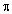.

2) Change of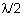in the path difference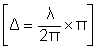3) No change in the nature of wave.

4) Compression is reflected as compression and rarefaction as a rarefaction.

5) Node is always formed at rigid surface

6) Direction of wave is changed.

(b) Reflection from rare medium:

1) No change in the phase.

2) No change in the path difference

3) No change in the nature of wave.

4) Compression is reflected as rarefaction while rarefaction as compression.

5) Antinode is formed at the surface of a rare medium.

6) Direction of the wave is changed.

### 12) stationary waves:

1) This is the wave produced by the superposition of two identical waves traveling along the same straight line but in opposite direction.

2) Energy is not transferred by these waves in the medium. It is only redistributed.

3) If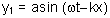be the propagation wave along +ve x-axis and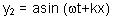be the progressive wave along –ve x-axis, then for the resultant stationary wave.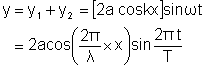These are shown in the following figure: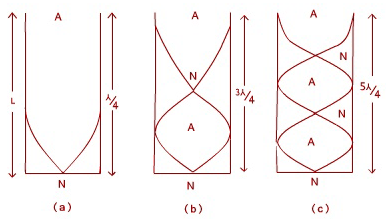Fig (5)

(a)   Fundamental node or first harmonic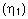.

(b)   First overtone or third harmonic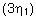.

(c)   Second overtone or fifth harmonic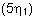.

Therefore the ratio of overtone is 3:5:7.

(e) It is clear that the only odd harmonics are produced in this pipe: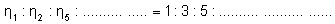2) Vibration of an open pipe:

(a) These pipes are open at both ends where antinodes are formed. At these ends compression is reflected as rarefaction while rarefaction as compression. Here the number of antinodes is more than that of nodes.

(b) For a pipe of length L, the frequency of fundamental node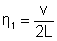and wavelength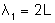First overtone or second harmonic frequency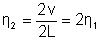, wavelength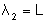Second overtone or third harmonic frequency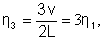wavelength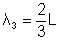These are shown in the following figure: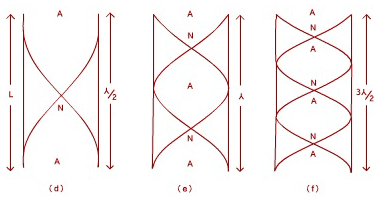Fig (6)

(a)   Fundamental node or first harmonic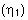(b)   First overtone or second harmonic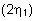(c)   Second overtone or third harmonic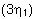Clearly in open pipe all the harmonics are produced. The ratio of the frequencies is: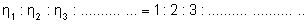In this condition the ratio of overtone is 2:3:4:5: …………

End correction: e = 0.6r, where ‘r’ is the radius of pipe. Therefore for a closed pipe the effective length of the air column = L+e = L + 0.6r.

For open pipe the effective length of the air column is L+2e = L+1.2r.

For a closed pipe with end correction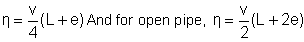By comparison: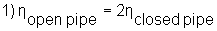(For fundamental only)

2) In open pipe all the harmonic are obtained while in closed pipe only odd harmonics are obtained.

3) The sound produced in open organ tube is pleasing and that of the closed organ tube is less pleasing.

### 18) resonance tube:

Resonance: If the frequency of a tuning fork used is same as the frequency of vibration of air column in the tube, then the intensity of sound becomes maximum. This is said to be the condition of resonance.

It is an example of a closed pipe in which the length of the air column can be changed by adjusting the level of water.

Application:

To determine the velocity of sound and the frequency of tuning fork

Formula:

If the first and second resonance lengths are L1 and L2 then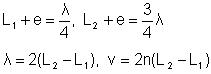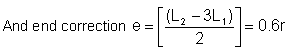19)

(a) Velocity of sound (Longitudinal wave) in elastic medium: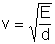Where E = Coefficient of elasticity of medium, d = density

For a solid medium: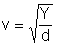, where E=Y = Young’s coefficient of elasticity

In a liquid medium: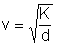, where E=K= Coefficient of volume elasticity.

In gaseous medium: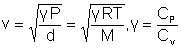M = molecular, P = pressure, T = temperature.

(b) In stretched vibrating wire or a string, the velocity of a transverse wave:### A few Basic Problems with Solutions on Sound Waves.

1. The velocity of sound is maximum in :

(a) C02

(b) S02

(c) NH3

(d) CH4

Ans: d) CH4, because the mass of methane is the least of the above options.

2. Velocity of sound waves in air is 330 m/s. For a particular sound in air, a path difference of 40 cm is equivalent to a phase difference of 1.6 . The frequency of this wave is:

(a) 165 Hz

(b) 150 Hz

(c) 660 Hz

(d) 330 Hz

Ans.

v = 330 m/s

= 1.6 .

x = 0.4 m

Now,     = 2x /

and, we know, that velocity is the product of frequency and wavelength.

Hence,  we get c) as the answer.

3. A sinusoidal wave travelling in the positive x direction has an amplitude of 15 cm, a wavelength of 40 cm and a frequency of 8 Hz. The vertical displacement of the medium at t = 0 and x = 0 is also 15 cm. (a) Find the angular wavenumber, period, angular frequency, and speed of the wave.

Ans:

= 2/T = 50.3 rad and, T = 1/f

Also, we know v = f = 320 cm/s.

4. Two sinusoidal waves of the same frequency travel in the same direction along a string. If A1 = 3cm, A2 = 4 cm and their respective phases are 0 and 90 degrees,what is the amplitude of the resultant wave?

Ans:

Resultant amplitude = (32+42+ 2 * 3 * 4 * cos 90)^0.5 = 5 cm.

5. A taut string for which = 0.005 kg/m under a tension of 80 N. How much power must be supplied to the string to generate sinusoidal waves at a frequency of 60 Hz and amplitude of 6 cm?

Ans:

v = T/=40 m/s.

= 2f.

Thus, having  P = 0.5 2A^2F/v = 512 W.

6. A wave of wavelength 4 mm is produced in air and it travels a speed of 300 m/s. Will it be audible?

Ans:

Frequency is  f = v/ =  300/0.004 = 75000 Hz.

This is much above audible range. It is ultrasonic wave.

7. A sound wave of amplitude 40 cm travels in air. If the difference between the maximum and minimum pressure at a given point is 2 mPa, find the amplitude of vibration of the particles of the medium. The bulk modulus of air is 0.14 MPa.

Ans:

Pressure amplitude = 2 mPa/2 = 1 mPa.

The displacement amplitude s0 = p/Bk .

Using  k = 2/, we get s0 = 6.6 A.

### Medium Difficulty Questions

1. For a person with normal hearing, the faintest sound that can be heard at a frequency of 400Hz has a pressure amplitude of 60Pa what will be the corresponding intensity. Take the speed of sound in air 344 m/s and density of air is 1.2 kg/m^3.

Ans:

I = P/A = 0.5 v (P)2/ B

Substituting B = v2

We hence reduce I = 4.4 * 10^-12 W/m^2.

2. Two identical machines are positioned in the same distance from a worker. The intensity of the sound delivered by each machine at the location of the worker is 0.2 W/m^2. Find the sound level heard by the worker (a) when one machine is operating and (b) when both machines are operating.

Ans: (a) The sound level at the location of the worker with 1 machine operating can be calculated from the Doppler Equation.

= 10 log (I/I0)

Thus,  = 10 log (2 * 10^-7/10^-12) = 53 dB.

(b)When both the machines are operating, the intensity is doubled to 4 * 10^-7 W/m^2, therefore the sound level is now:

=  10 log (4 * 10^-7)/(10^-12) = 56 dB

From these results, we see that when the intensity is doubles, the sound level increases by only 3 dB.

3. As an ambulance travels east down a highway at a speed of 33.5 m/s (75 mi/h) its siren emits sound at a frequency of 400Hz. What frequency is heard by a person in a car travelling west at 24.6 m/s (55 mi/h)

(a) as the car approaches the ambulance and

(b) as the car moves away from the ambulance.

Ans:

We can use the Doppler equation in both cases, taking the speed of sound in air to be 343 m/s. As the ambulance and car approach each other, the person in the car hears the frequency,

f1 = (343 + 24.6)/ (343 - 33.5) = 475 Hz.

f2 = (343 - 24.6)/ (343 + 33.5) = 338 Hz.

The change in frequency detected by the person in the car is 475 - 338 = 137 Hz, which is more than 30 % of the true frequency.

4. Estimate how far away a cicada can be heard if the lowest possible audible intensity of a sound it produces is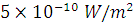and the power of the cicada's sound source is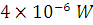..

Ans :We can estimate that the total power of the cicada's sound is distributed uniformly over the spherical surface of radius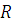. Then at distancethe intensity of the sound isThe largest radius is achieved when the intensity is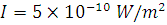. Then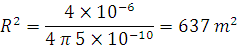Then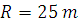### Some Questions to solve Independently:

1. Two waves y1 = A cos( 0.5 πx − 100 πt)

and  y2 = A cos( 0.46  πx − 92  πt) are travelling in a pipe placed along x-axis. Find the number of times

intensity is maximum in time interval of 1 sec.

(A) 4

(B) 6

(C) 8

(D) 10

Ans: (A)

|f1 − f2| = 4 Hz

2.  Find wave velocity of louder sound

(A) 100 m/s

(B) 192 m/s

(C) 200 m/s

(D) 96 m/s

Ans. (C)

v1 = v2 = 200 m/s

3. Find the number of times y1+ y2 = 0 at x = 0 in 1 sec

(A) 100

(B) 46

(C) 192

(D) 96

Sol. (D)

y1 + y2 = A cos 100πt + A cos 92π t = 0

cos 100πt = −cos 92πt

100πt = (2n + 1)π − 92πt

t = (2n+1)/192

∆t = tn+1 − tn = 2/192